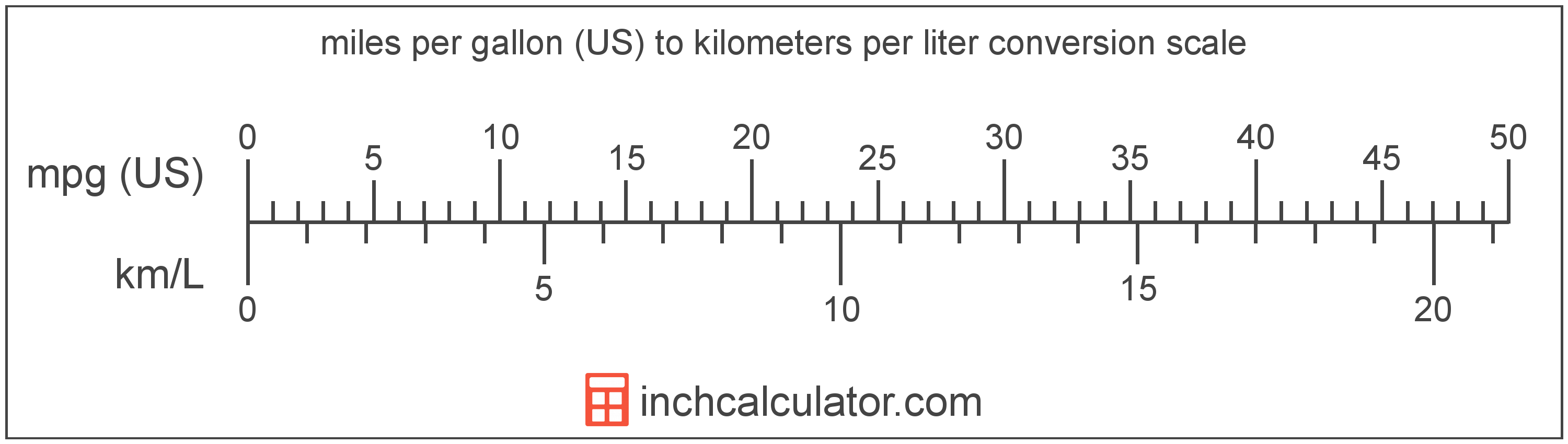# Kilometers per Liter to Miles per Gallon (US) Converter

Enter the fuel economy in kilometers per liter below to get the value converted to miles per gallon (US).

Results in Miles per Gallon (US):1 km/L = 2.352145 mpg (US)

Do you want to convert miles per gallon (US) to kilometers per liter?

## How to Convert Kilometers per Liter to Miles per Gallon (US)

To convert a measurement in kilometers per liter to a measurement in miles per gallon (US), multiply the fuel economy by the following conversion ratio: 2.352145 miles per gallon (US)/kilometer per liter.

Since one kilometer per liter is equal to 2.352145 miles per gallon (US), you can use this simple formula to convert:

miles per gallon (US) = kilometers per liter × 2.352145

The fuel economy in miles per gallon (US) is equal to the fuel economy in kilometers per liter multiplied by 2.352145.

For example, here's how to convert 5 kilometers per liter to miles per gallon (US) using the formula above.
miles per gallon (US) = (5 km/L × 2.352145) = 11.760725 mpg (US)Kilometers per liter and miles per gallon (US) are both units used to measure fuel economy. Keep reading to learn more about each unit of measure.

## What Are Kilometers per Liter?

Kilometers per liter is the distance that can be traveled in kilometers using one liter of fuel.

Kilometers per liter can be abbreviated as km/L, and are also sometimes abbreviated as kpl or kmpl. For example, 1 kilometer per liter can be written as 1 km/L, 1 kpl, or 1 kmpl.

In the expressions of units, the slash, or solidus (/), is used to express a change in one or more units relative to a change in one or more other units.

## What Are Miles per Gallon (US)?

Miles per gallon is the distance traveled in miles consuming just one gallon of fuel.

When evaluating fuel efficiency, the more miles per gallon, the more fuel efficient a vehicle is and the less fuel consumed. Thus, the higher the mpg rating of a vehicle the less fuel it will consume.

Miles per gallon (US) can be abbreviated as mpg (US), and are also sometimes abbreviated as mpg. For example, 1 mile per gallon (US) can be written as 1 mpg (US) or 1 mpg.

## Kilometer per Liter to Mile per Gallon (US) Conversion Table

Table showing various kilometer per liter measurements converted to miles per gallon (US).
Kilometers Per Liter Miles Per Gallon (US)
1 km/L 2.3521 mpg (US)
2 km/L 4.7043 mpg (US)
3 km/L 7.0564 mpg (US)
4 km/L 9.4086 mpg (US)
5 km/L 11.76 mpg (US)
6 km/L 14.11 mpg (US)
7 km/L 16.47 mpg (US)
8 km/L 18.82 mpg (US)
9 km/L 21.17 mpg (US)
10 km/L 23.52 mpg (US)
11 km/L 25.87 mpg (US)
12 km/L 28.23 mpg (US)
13 km/L 30.58 mpg (US)
14 km/L 32.93 mpg (US)
15 km/L 35.28 mpg (US)
16 km/L 37.63 mpg (US)
17 km/L 39.99 mpg (US)
18 km/L 42.34 mpg (US)
19 km/L 44.69 mpg (US)
20 km/L 47.04 mpg (US)
21 km/L 49.4 mpg (US)
22 km/L 51.75 mpg (US)
23 km/L 54.1 mpg (US)
24 km/L 56.45 mpg (US)
25 km/L 58.8 mpg (US)
26 km/L 61.16 mpg (US)
27 km/L 63.51 mpg (US)
28 km/L 65.86 mpg (US)
29 km/L 68.21 mpg (US)
30 km/L 70.56 mpg (US)
31 km/L 72.92 mpg (US)
32 km/L 75.27 mpg (US)
33 km/L 77.62 mpg (US)
34 km/L 79.97 mpg (US)
35 km/L 82.33 mpg (US)
36 km/L 84.68 mpg (US)
37 km/L 87.03 mpg (US)
38 km/L 89.38 mpg (US)
39 km/L 91.73 mpg (US)
40 km/L 94.09 mpg (US)